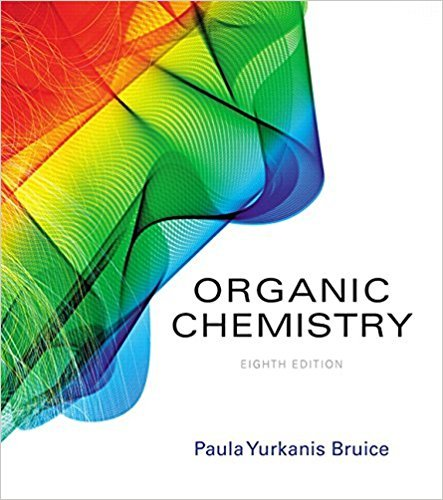×
×

# Solved: The observed rotation of 2.0 g of a compound in 50 mL of solution in aISBN: 9780134042282 482

## Solution for problem 33 Chapter 4.11

Organic Chemistry | 8th Edition

• Textbook Solutions
• 2901 Step-by-step solutions solved by professors and subject experts
• Get 24/7 help from StudySoup virtual teaching assistantsOrganic Chemistry | 8th Edition

4 5 1 267 Reviews
19
3
Problem 33

The observed rotation of 2.0 g of a compound in 50 mL of solution in a polarimeter tube 20 cm long is+138. What is the specific rotation of the compound?

Step-by-Step Solution:
Step 1 of 3

Chemistry Notes Week 12 Chapter 8: Chemical Reactions A chemical equation uses chemical symbols to denote what occurs in a chemical reaction A. NH3 + HCl —> NH4Cl B. Ammonia and Hydrochloride reaction to form ammonium chloride Labels (indicate the physical state) A. Gas (g) B. Liquid (l) C. Solid (s) D. Aqueous (aq)- dissolved in water...

Step 2 of 3

Step 3 of 3

##### ISBN: 9780134042282

This full solution covers the following key subjects: . This expansive textbook survival guide covers 127 chapters, and 2116 solutions. Organic Chemistry was written by and is associated to the ISBN: 9780134042282. This textbook survival guide was created for the textbook: Organic Chemistry, edition: 8. The full step-by-step solution to problem: 33 from chapter: 4.11 was answered by , our top Chemistry solution expert on 03/16/18, 04:59PM. The answer to “The observed rotation of 2.0 g of a compound in 50 mL of solution in a polarimeter tube 20 cm long is+138. What is the specific rotation of the compound?” is broken down into a number of easy to follow steps, and 30 words. Since the solution to 33 from 4.11 chapter was answered, more than 710 students have viewed the full step-by-step answer.

Unlock Textbook Solution